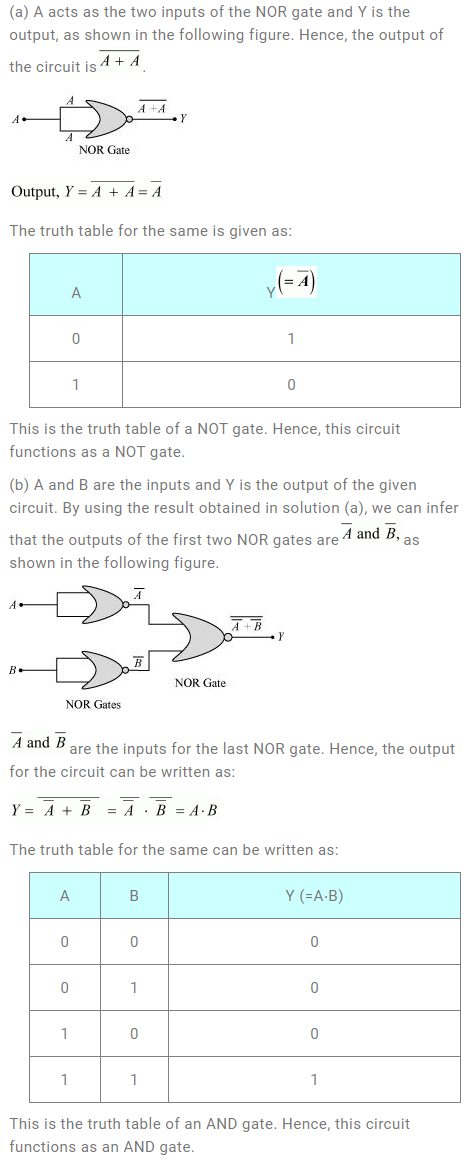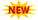SSC Phase 10 Notification || TSSPDCL AE Notification || PPSC Naib Tehsildar Admit Card ||

# NCERT Solutions For Class 12 Physics Chapter 14 Semiconductors Electronics: Materials, Devices And Simple Circuits

NCERT Solutions For Class 12 Physics Chapter 14 deals with the exercise problems based on Semiconductors, Energy Gap, Valence bands, conduction bands, junction diodes, triodes, vacuum tubes, etc. Reading the chapter thoroughly and solving the exercise problems enables the reader to understand the topic clearly. If they need help solving any problem in this lesson, they can refer to the Chapter 14 Semiconductors Class 12 NCERT Solutions article we have provided here. All Exercise Problems at the end of all chapters are provided in NCERT Solutions For Class 12 Physics. This can help candidates get clear understanding of concepts provided in the chapters. These form from a very basic level and we suggest students read them carefully before attempting to solve any exercise problems.

## NCERT Class 12 Physics Chapter 14 Solutions

Subtopics in the NCERT Solutions For Class 12 Physics Chapter 14 are mentioned below.

 Section Topic Name 14 Semiconductors 14.1 Introduction 14.2 Classification of Metals, Conductors, and Semiconductors 14.3 Intrinsic Semiconductor 14.4 Extrinsic Semiconductor 14.5 p-n Junction 14.6 Semiconductor Diode 14.7 Application of Junction Diode as a Rectifier 14.8 Special Purpose p-n Junction Diodes 14.9 Junction Transistor 14.10 Digital Electronics and Logic Gates 14.11 Integrated Circuits

### NCERT Class 12 Physics Chapter 14 Solutions

Question 14.1: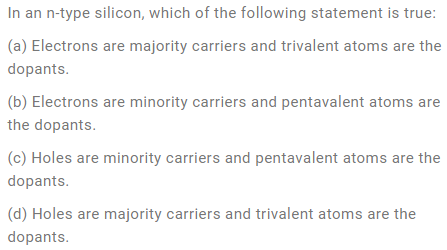Solution: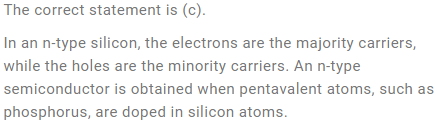Question 14.2: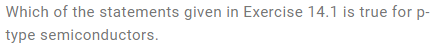Solution: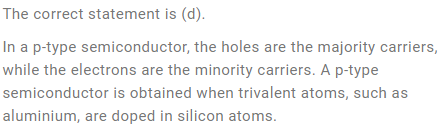Question 14.3: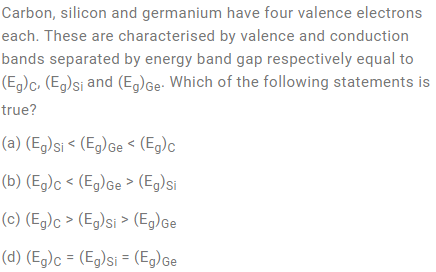Solution: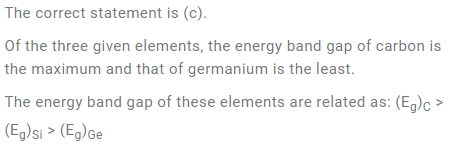Question 14.4: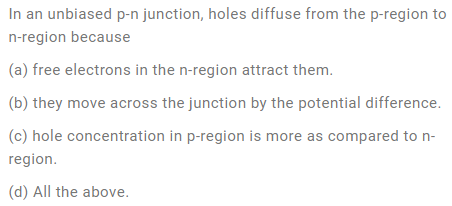Solution: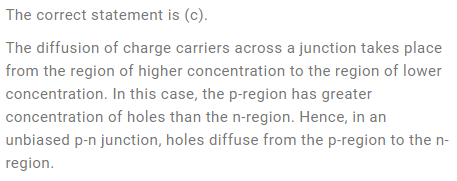Question 14.5: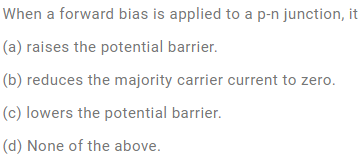Solution: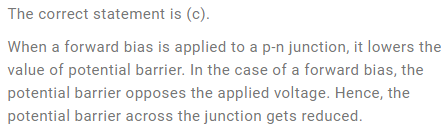Question 14.6: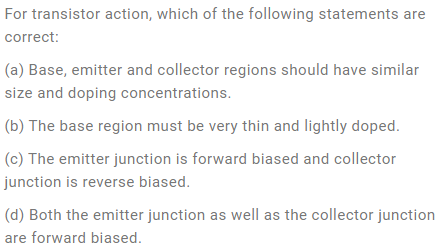Solution: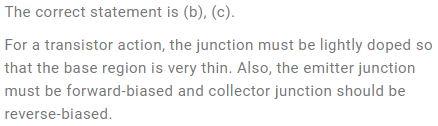Question 14.7: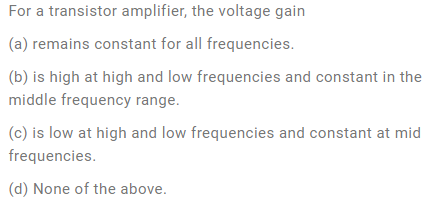Solution: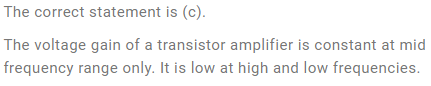Question 14.8: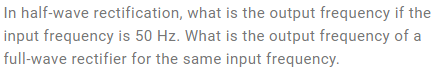Solution: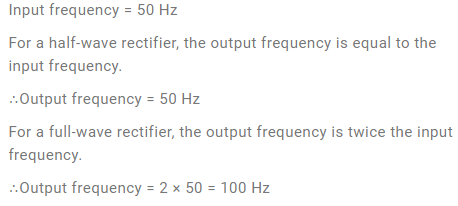Question 14.9: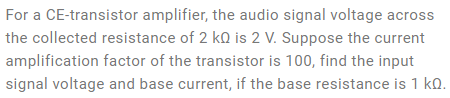Solution: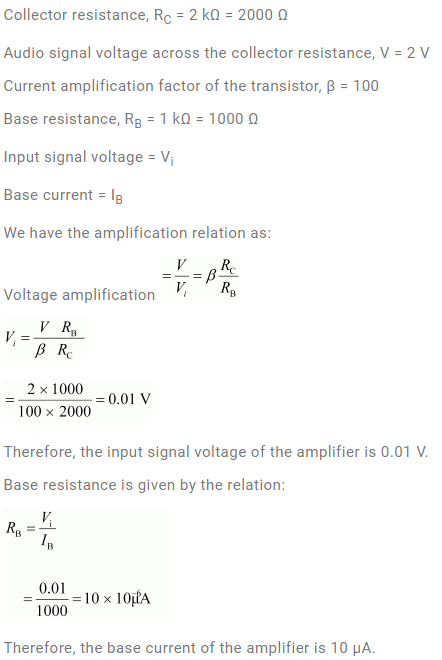Question 14.10: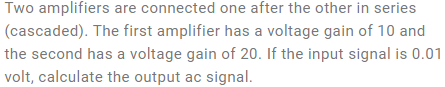Solution: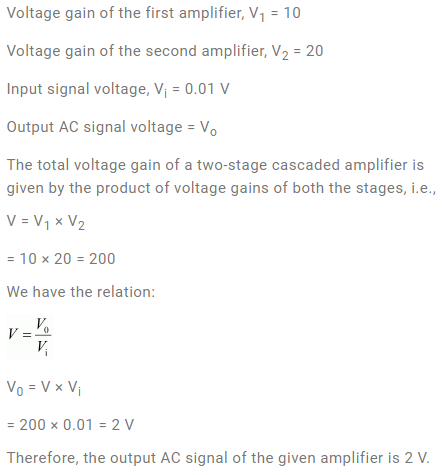Question 14.11: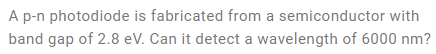Solution: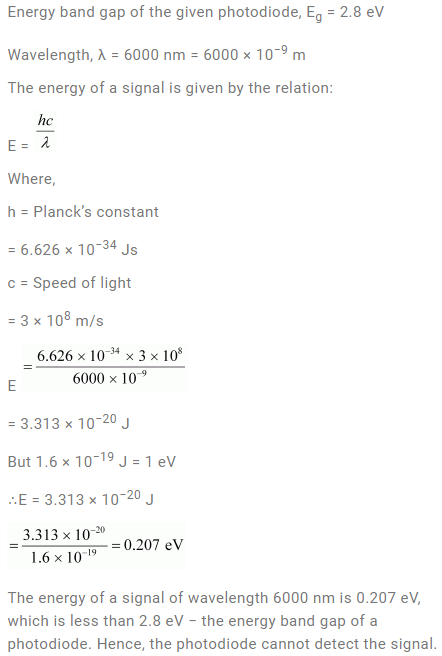Question 14.12: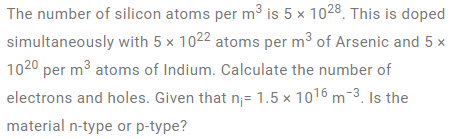Solution: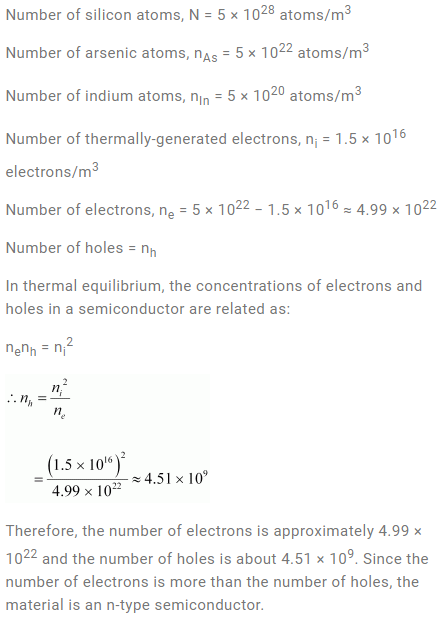Question 14.13: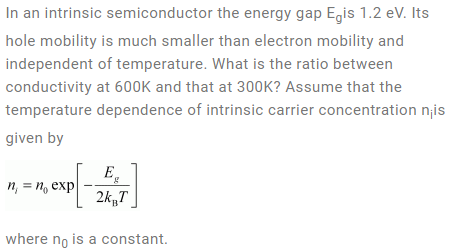Solution: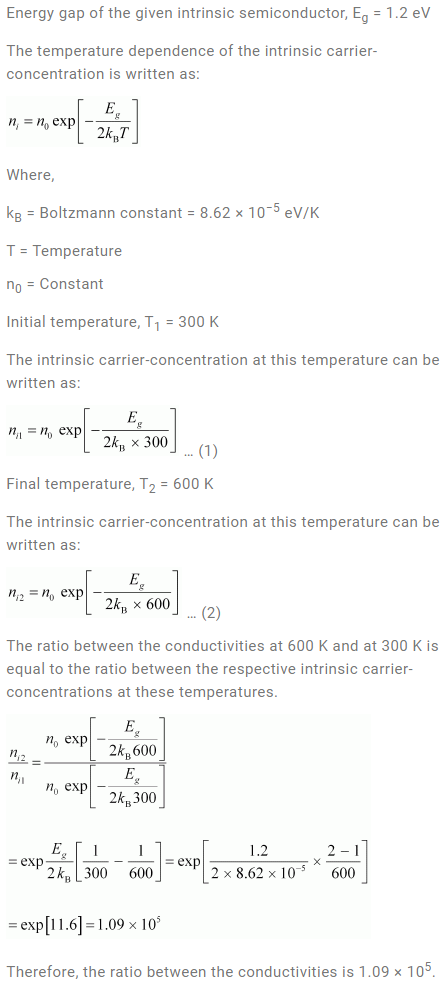Question 14.14: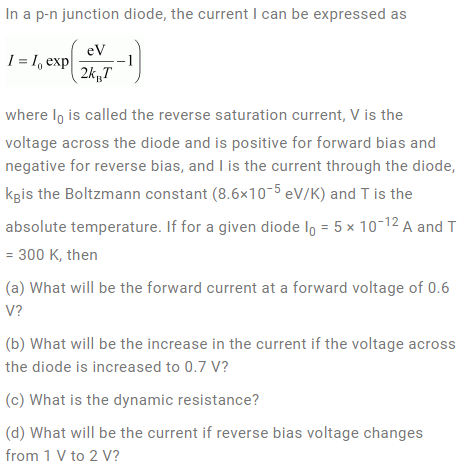Solution: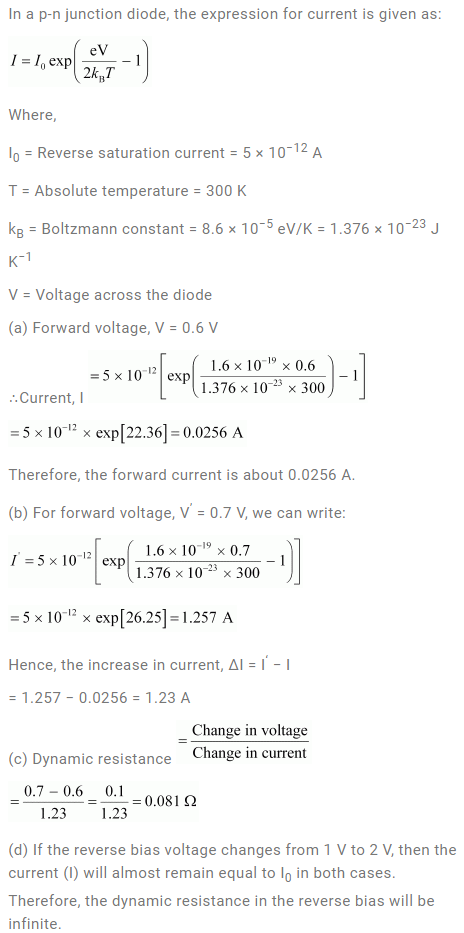Question 14.15: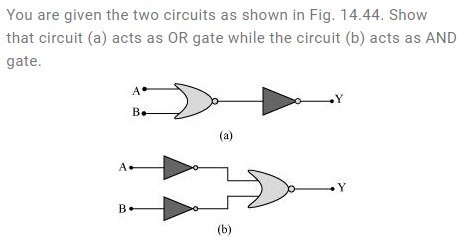Solution: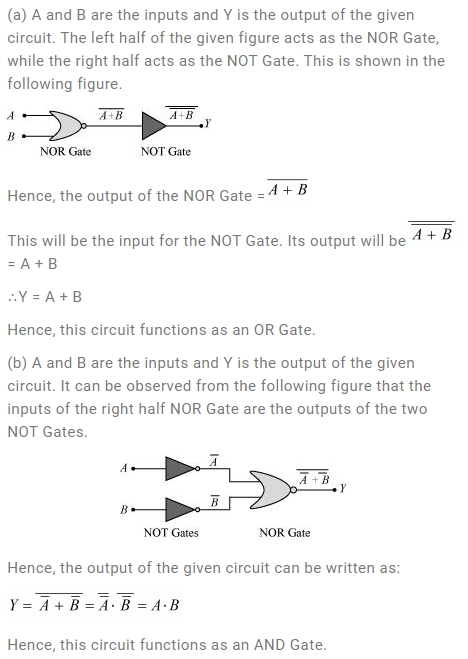Question 14.16: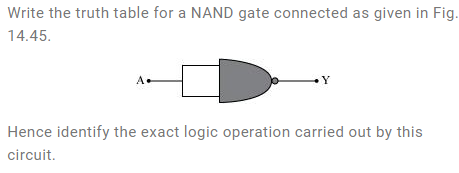Solution: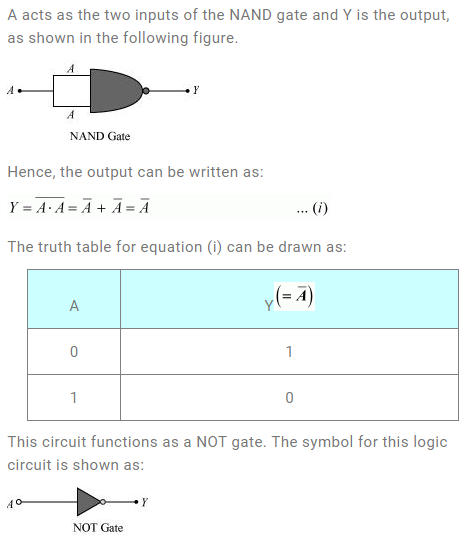Question 14.17: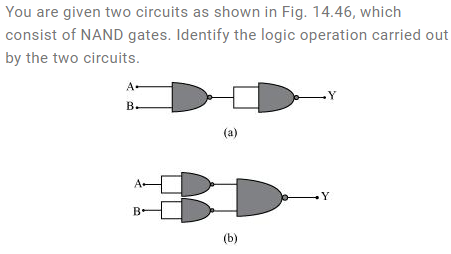Solution: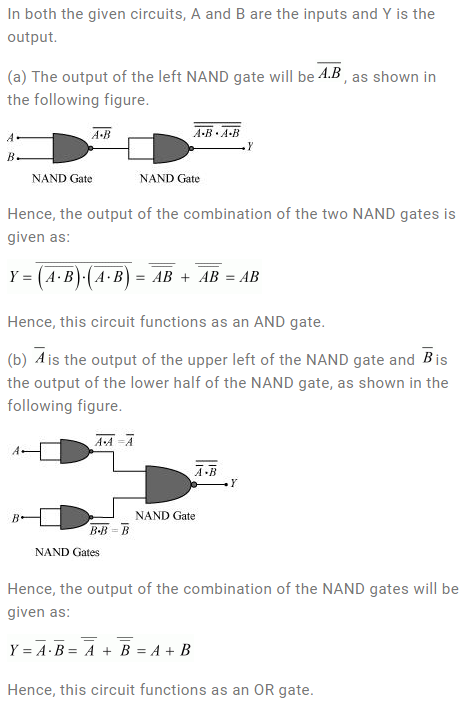Question 14.18: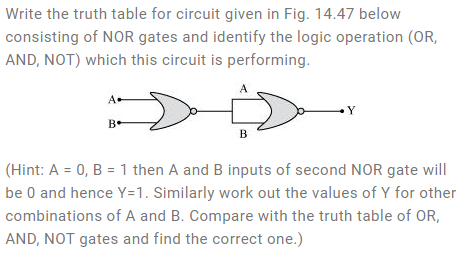Solution: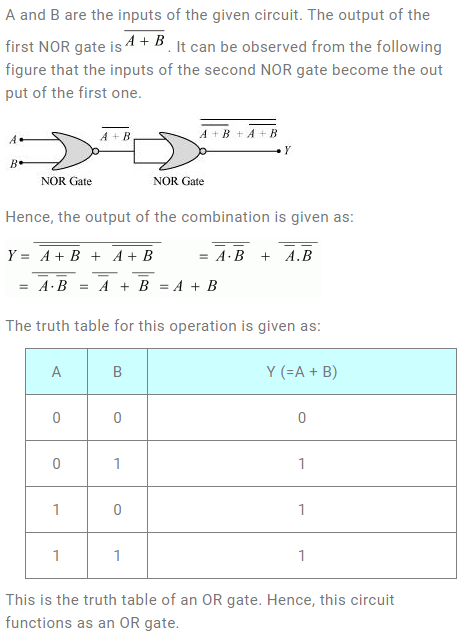Question 14.19: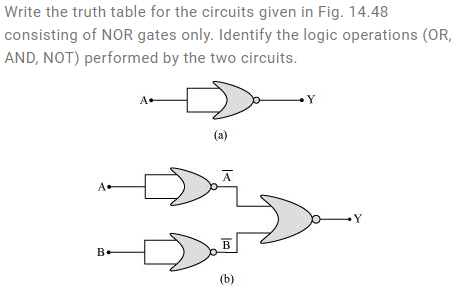Solution: